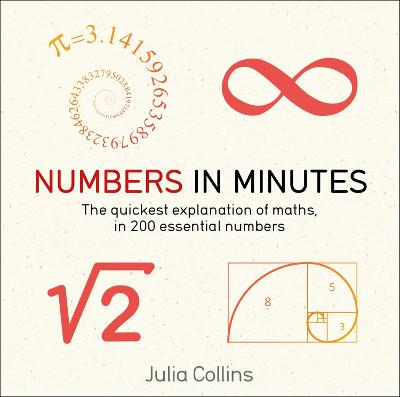•# Numbers in Minutes - In Minutes (Paperback)

(author)
£10.99
Paperback 416 Pages
Published: 07/11/2019
• In stock

Usually dispatched within 48 hours

Why 60 seconds in a minute? Who invented zero? What exactly is pi? Why do mathematicians hunt prime numbers? And how can you get a number bigger than infinity?

To find out, take a tour through 200 important, fascinating and unusual numbers - the easy and entertaining way to grasp mathematics.

Numbers in Minutes demystifies the maths surrounding the key numbers including: zero, 1-40, negatives, percentages, prime numbers, fractions, decimals, pi, exponentials, imaginary numbers, squares and cubes, roots and powers, Fibonacci numbers, the golden ratio, millions and trillions, a googol, 'perfect,' 'kissing,' 'vampire' and 'weird' numbers, infinity, infinity+1 and other sizes of infinity...

Every number is explained in a few short paragraphs with a helpful picture, making the maths simple to understand and remember.

Publisher: Quercus Publishing
ISBN: 9781787477315
Number of pages: 416
Weight: 360 g
Dimensions: 126 x 126 x 30 mm